## STATSFILT

 Various statistical filters primarily used to reduce noise in an image

 USAGE: statsfilt [-s statistic] [-p power] infile outfile USAGE: statsfilt [-h or -help] Option -s ...... statistic ... id number for the statistical filter to compute; default=1 ....................... statistic=1: simple average (arithmetic mean) of neighborhood ..................................... pixels ....................... statistic=2: minimum value of neighborhood pixels ....................... statistic=3: maximum value of neighborhood pixels ....................... statistic=4: average of the minimum and maximum values ....................... statistic=5: arithmetic mean excluding min and max values ....................... statistic=6: standard deviation of neighborhood pixels ....................... statistic=7: geometric mean of neighborhood pixels ....................... statistic=8: harmonic mean of neighborhood pixels ....................... statistic=9: Lp mean of neighborhood pixels; .................................... requires p=integer option ....................... statistic=10: contraharmonic mean of neighborhood pixels; ..................................... require p=integer option ....................... statistic=11: linear distance weighted average of ..................................... neighborhood pixels, excluding the center ....................... statistic=12: inverse distance weighted average of ..................................... neighborhood pixels, excluding the center ....................... statistic=13: minimum or maximum whichever value is closest ..................................... to center ....................... statistic=14: average (arithmetic mean) without the center pixel -p ...... power ....... exponent value to use with statistic 9 and 10; ....................... exponent=integer; default=2 -h ...... get help -help ... get help PURPOSE: To compute various localized (neighborhood) statistical filters on an image. DESCRIPTION: STATSFILT is a collection of localized (neighborhood) statistical filters most of which are used for mostly for noise reduction in an image. Note that most noise reduction filters trade noise reduction for some degree of blurring. Arguments: -h or help ..... displays help information. -s ..... statistic ..... statistic is a numerical id for the type of statistic to be calculated. A value of 1 replaces each input pixel with the simple average (or arithmetic mean) of all the pixels in the 3x3 neighborhood about that input pixel. A value of 2 replaces the input pixel with the minimum value found in the neighborhood. A value of 3 replaces the input pixel with the maximum value found in the neighborhood. A value of 4 replace the input pixel with average of the minimum and maximum values in the neighborhood. A value of 5 replaces the input pixel with simple average of all the pixels in the neighborhood, except the minimum and maximum values. Thus it is throwing out the two extreme outliers. A value of 6 replaces the input pixel with the standard deviation of all the pixels in the neighborhood. This is more of an edge operator that a noise reduction operator. A value of 7 replaces the input pixel with geometric mean of all the pixels in the neighborhood. The geometric mean is computed by taking the ln of each neigborhood pixel value, averaging these values and then taking the exp of the result. The geometric mean is used especially for noise reduction when dealing with one-sided noise that is all bright spikes (but not well at all dark spikes). A value of 8 replaces the input pixel with the harmonic mean of all the pixels in the neighborhood. The harmonic mean is computed by taking the inverse of each pixel value, averaging these results and then taking the inverse of the result. The harmonic mean is used especially for noise reduction when dealing with one-sided noise that is all bright spikes (but not well at all dark spikes). A value of 9 replaces the input pixel with the Lp mean of all the pixels in the neighborhood. The Lp mean is computed by raising each pixel value in the neighborhood to some power, averaging these results and then raising the result to the equivalent inverse power (i.e. the negative value of the power). A choice of p=0 is not allowed. Typically p is a low valued integer, i.e. 2 or -2. Note that p=1 is equivalent to the simple average (arithmetic mean) and p=-1 is equivalent to the harmonic mean. The Lp mean is used especially for noise reduction when dealing with one-sided noise that is either all bright spikes or all dark spikes. For all bright spikes, use negative p values and for all dark spikes, use positive p values. A value of 10 replaces the input pixel with the contraharmonic mean of all the pixels in the neighborhood. The contraharmonic mean is computed by taking the average of all the pixels in the neighborhood raised to the power p+1 and dividing that by the average of all the pixels in the neighborhood raised to the power p. The power p can be any integer. A choice of p=-1 is not allowed. Note that p=0 is equivalent to the simple average (arithmetic mean). The contraharmonic mean is used especially for noise reduction when dealing with one-sided noise that is either all bright spikes or all dark spikes. For all bright spikes, use negative p values and for all dark spikes, use positive p values. A value of 11 replaces each pixel in the input image with a linear distance weighted average of all pixels in the neighborhood, except the original center pixel. Weighting is measured as one minus the absolute difference between the graylevel of each neighbor and the center pixel. Thus pixels with smaller differences (i.e. values closer to that of the the center pixel) are weighted higher. A value of 12 replaces each pixel in the input image with an inverse distance weighted average of all pixels in the neighborhood, except the original center pixel. Weighting is measured as inverse of the absolute difference between the graylevel of each neighbor and the center pixel. Thus pixels with smaller differences (i.e. values closer to that of the the center pixel) are weighted higher. A value of 13 replaces each pixel in the input image with either the minimum or maximum value of all pixels in the neighborhood, whichever is closest in value to the given (center) pixel. This produces a sharpening effect on the image. A value of 14 replaces each pixel in the input image with the average of all the pixels in the neighborhood, but the center pixel. All of these statistical filters are computed within a 3x3 neighborhood only. As it is, some of the latter statistics take a very long time to compute as this script is based up the use of fx. -p ..... power ..... exponent value to use to raise the pixel value to the given power. The values may be positive or negative integers. The default is 2 WARNING: Due to the use of -fx, some of these filters are slow. On a Macintosh PowerPC G4, 1.42GHz with 512 Mbytes RAM and an image size of 128x128 pixels, it takes only a few seconds to run filters 1-10, 13 and 14, but about 20 seconds for filters 11 and 12. CAVEAT: No guarantee that this script will work on all platforms, nor that trapping of inconsistent parameters is complete and foolproof. Use At Your Own Risk.

### EXAMPLES

Some General Statistical Filters

 Original Image Arithmetic Mean(Average) Arguments: -s 1 Minimum Arguments: -s 2 Maximum Arguments: -s 3Average of Min and Max Arguments: -s 4 Standard Deviation Arguments: -s 6 (and -normalize) Min or Max Whichever Value Is Closest Arguments: -s 13 (sharpening effect)Filtering Of Bright and Dark Noise

 Noisy Image Arithmetic Mean Arguments: -s 1 Arithmetic Mean without min and max Arguments: -s 5Geometic Mean Arguments: -s 7 Harmonic Mean Arguments: -s 8 Lp Mean Arguments: -s 9 -p 2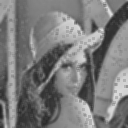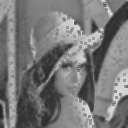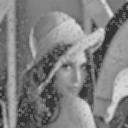Contraharmonic Mean Arguments: -s 10 -p 1 Linear Distance Weighted Average Arguments: -s 11 Inverse Distance Weighted Average Arguments: -s 12Filtering Of Bright Only Noise

 Noisy Image Arithmetic Mean Arguments: -s 1 Arithmetic Mean without min and max Arguments: -s 5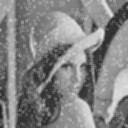Geometic Mean Arguments: -s 7 Harmonic Mean Arguments: -s 8 Lp Mean Arguments: -s 9 -p -2Lp Mean Arguments: -s 9 -p -3 Contraharmonic Mean Arguments: -s 10 -p -2Filtering Of Dark Only Noise

 Noisy Image Arithmetic Mean Arguments: -s 1 Arithmetic Mean without min and max Arguments: -s 5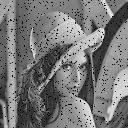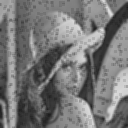Lp Mean Arguments: -s 9 -p 2 Lp Mean Arguments: -s 9 -p 3 Contraharmonic Mean Arguments: -s 10 -p 1Comparison To Other Methods For Dense Bright Noise

 Noisy Image Arithmetic Mean Arguments: -s 1 Arithmetic Mean without min and max Arguments: -s 5 Arithmetic Mean linear distance wts Arguments: -s 11 Arithmetic Mean inverse distance wts Arguments: -s 12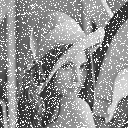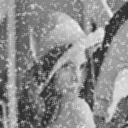Geometic Mean Arguments: -s 7 Harmonic Mean Arguments: -s 8 Lp Mean Arguments: -s 9 -p -2 Lp Mean Arguments: -s 9 -p -6 Contraharmonic Mean Arguments: -s 10 -p -2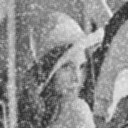Median -median 1 Median Second Iteration Median Third Iteration IM Noise Removal -noise 1 IM Noise Removal Second InterationWhat the script does is as follows: Access the 9 pixels in a 3x3 neighborhood of each pixel in the image. Uses -fx to compute various kinds of statistical averages. Outputs the computed statistic at each corresponding output pixel. This is equivalent to the following IM commands for the case of arguments: -s 5 (the average without the min and max values). pixels="aa=p[-1,-1]; ab=p[0,-1]; ac=p[+1,-1]; ba=p[-1,0]; \ bb=p[0,0]; bc=p[+1,0]; ca=p[-1,+1]; cb=p[0,+1]; cc=p[+1,+1];" tot="((aa)+(ab)+(ac)+(ba)+(bb)+(bc)+(ca)+(cb)+(cc))" min="min(min(min(min(min(min(min(min(aa,ab),ac),ba),bb),bc),ca),cb),cc)" max="max(max(max(max(max(max(max(max(aa,ab),ac),ba),bb),bc),ca),cb),cc)" convert \$infile -fx "\$pixels u=(\$tot-\$max-\$min)/7" \$outfile